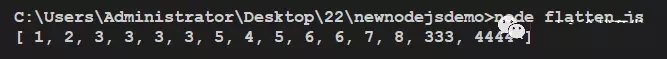• python判断一个数组是否为空
万次阅读
2018-08-13 11:59:12

第一种方案（适用于前端传过来数据判定）

mystr = ''

if mystr:
list = mystr.split('*')
print len(list)
print list
else:
print 0

第二种方案(适用于自己定义的数据，[]为空是默认为False)

mylist = []

if mylist:
print 'yes'
else:
print 'no'

第三种方案（适用于字符数组，非字符数组会报错）

mystr = '[]'

mylist = eval(mystr)

if mylist:
print 'yes'
else:
print 'no'

更多相关内容
• 在Java程序设计里面，相信大部分人都知道如何判断一个一维数组是否为空，示例如下：public int primeNumberCount(int[] array){ if(array==null||array.length==0) return 0; 那么在二维数组中，又如何判断二维...

在Java程序设计里面，相信大部分人都知道如何判断一个一维数组是否为空，示例如下：
if(array==null||array.length==0)
return 0;
那么在二维数组中，又如何判断二维数组为空呢？在判断之前，我们要先了解如何获取二维数组的行数和列数，示例如下：

public boolean Find(int target, int[][] array) {
int rows = array.length;//行数
int columns = array.length;//列数
接下来进入主题，要如何判断二维数组是否为空呢？Java中判断二维数组是否为空，要判断三种情况：

1、二维数组首地址是否为空，即array==null；

2、二维数组是否为{}，即array.length==0的情况；

3、二维数组是否为{{}}，即array.length=1&&array.length==0的情况；

综上所述，Java中判断二维数组为空的条件为：

if((array==null||array.length==0)||(array.length==1&&array.length==0))---------------------
作者：XuTong_Zhang
来源：CSDN
原文：https://blog.csdn.net/m0_37451060/article/details/80143831

转载于:https://www.cnblogs.com/greatLong/p/10540048.html

展开全文• 前言：判断数组为空，是一个常见用法。Python与Java的方法不同，需区分 Python–方法： 1.根据长度判断 长度0时，表示。（其中”判断条件”成立时（非零），则执行后面的语句） lst = [] if len(lst): ...

前言：判断数组为空，是一个常见用法。Python与Java的方法不同，需区分

Python–方法：
1.根据长度判断
长度为0时，表示空。（其中”判断条件”成立时（非零），则执行后面的语句）

lst = []
if len(lst):
print 'c'
else:
print 'cc'

2.根据逻辑判断
由于一个空 list 本身等同于 False

lst = []
if lst:
print 'c'
else:
print 'cc'

注意：
两个以上的判断条件：
代码：

lst = []
if lst == 1 and lst:
print 'a'

此时执行，会提示 IndexError: list index out of range
需将if的两个条件互换，则不会报错

lst = []
if lst and lst == 1 :
print 'a'

Java–数组为空

  if(arr==null||arr.length==0)
return -1;
展开全文• php中经典方法实现判断多维数组是否为空复制代码 代码如下://判断一个数组是否为空/**array(); array(array(),array(),array()); array(array(),array(array(),array(1=>1)),array()); 非 */function is_...

php中经典方法实现判断多维数组是否为空

复制代码 代码如下:

//判断一个数组是否为空

/**

array(

); 空

array(

array(

),

array(

),

array(

)

); 空

array(

array(

),

array(

array(

),

array(

1=>1

)

),

array(

)

); 非 空

*/

function is_array_null($value) { if (empty($value))

{

return $value; } else { return is_array($value) ? array_map('array_null', $value) : addslashes($value);

}

}

时间： 2011-10-20

如下所示: 复制代码 代码如下: <?php /**  * 返回数组的维度  * @param  [type] $arr [description] * @return [type] [description] */function arrayLevel($arr){     $al = array(0); function aL($arr,&$al,$level=0){         if(is_array($arr)){$level++;

本文实例总结了php常用数组array函数.分享给大家供大家参考,具体如下: array_combine 功能:用一个数组的值作为新数组的键名,另一个数组的值作为新数组的值 案例: <?php $a = array("one","two","three");$b = array("一","二","三"); $c = array_combine($a,$b); print_r($c

复制代码 代码如下: <?php /** * 返回数组的维度 * @param  [type] $arr [description] * @return [type] [description] */function arrayLevel($arr){    $al = array(0); function aL($arr,&$al,$level=0){        if(is_array($arr)){$level++;            $al array_unique(PHP 4 >= 4.0.1, PHP 5) array_unique -- 移除数组中重复的值 说明 array array_unique ( array array ) array_unique() 接受 array 作为输入并返回没有重复值的新数组. 注意键名保留不变.array_unique() 先将值作为字符串排序,然后对每个值只保留第一个遇到的键名,接着忽略所有后面的键名.这并不意味着在未排序的 array 中同一个值的第一个出现的键名会被保留. 注: 当且 本文实例讲述了php判断数组中是否存在指定键(key)的方法.分享给大家供大家参考.具体分析如下: php中有两个函数用来判断数组中是否包含指定的键,分别是array_key_exists和isset array_key_exists语法如下 array_key_exists($key, $array) 如果键存在返回true isset函数语法如下 isset($array[$key]) 如果键存在返回true 演示代码如下: <?php$array = array("Zero&quo

HP没有内置判断是否索引数组的方法,简单实现了一个,用法: 复制代码 代码如下: echo is_assoc($array)?'索引数组':'不是索引数组'; is_assoc函数如下: 复制代码 代码如下: function is_assoc($array) {        if(is_array($array)) {$keys = array_keys($array); return$keys != array_keys($keys); 方法一:采用in_array(value,array,type) type 可选.如果设置该参数为 true,则检查搜索的数据与数组的值的类型是否相同. 复制代码 代码如下:$arr = array('可以','如何','方法','知道','沒有','不要');//in_array(value,array,type)$isin = in_array("如何2",$arr);if($isin){ echo "in====".$isin;}else{    ec

本文介绍了PHP开发中遇到的数组问题,这里介绍了判断PHP数组为空的5种方法,有需要的朋友可以借鉴参考一下. 1. isset功能:判断变量是否被初始化 说明:它并不会判断变量是否为空,并且可以用来判断数组中元素是否被定义过 注意:当使用isset来判断数组元素是否被初始化过时,它的效率比array_key_exists高4倍左右 <?php $a = '';$a['c'] = ''; if (!isset($a)) echo '$a 未被初始化' . ""; if (!iss

本文实例讲述了PHP实现判断数组是一维.二维或几维的方法.分享给大家供大家参考,具体如下: 这里使用的自定义函数,可以判断数组是一维的,还是二维的,或是几维的数组: function getmaxdim($vDim) { if(!is_array($vDim)) return 0; else { $max1 = 0; foreach($vDim as $item1) {$t1 = $this->getmaxdim($item1); if( $t1 >$max1) $max1 =$t1;本文实例讲述了PHP判断一个数组是另一个数组子集的方法.分享给大家供大家参考,具体如下: 前言 今天完成一个算法的过程中,有几个需求模块,其中就有判断$a数组是否是$b数组的子集,可能最近我写c比较多,直接就用for循环实现了,但是感觉代码量比较大,不够优雅!在qq群里集思广益了一下,发现很多php提供的系统功能函数都是可以供调用的,这里记录一下 需求 最少的时间复杂度判断$a数组是否是$b数组的子集 // 快速的判断$a数组是否是$b数组的子集 $a = array(135,138);$b如何判断两个数组相等呢?其实很简单,用 == 或者 === 就可以了 php手册里说明如下: 那像 array('k'=>array())这样的多维数组能用如上方法判断相等吗?当然也可以. 若数组是数字索引的,就要注意一下了,见代码: 复制代码 代码如下: <?php $a = array("apple", "banana");$b = array(1 => "banana", "0" => &quo

之前记录过JavaScript中判断为数字类型的多种方式,这篇看看如何判断为整数类型(Integer). JavaScript中不区分整数和浮点数,所有数字内部都采用64位浮点格式表示,和Java的double类型一样.但实际操作中比如数组索引.位操作则是基于32位整数. 方式一.使用取余运算符判断 任何整数都会被1整除,即余数是0.利用这个规则来判断是否是整数. 复制代码 代码如下: function isInteger(obj) {     return obj%1 === 0 } isIn

我这里总结了判断记录是否存在的常用方法: sql语句:select count(*) from tablename; 然后读取count(*)的值判断记录是否存在.对于这种方法性能上有些浪费,我们只是想判断记录记录是否存在,没有必要全部都查出来. 以下这个方法是我推荐的. sql语句:select 1 from tablename where col = col limit 1; 然后读取语句执行所影响的行数. 当然这里limit 1很重要.这要mysql找到一条记录后就不会在往下找了.这里执行

一.判断字符串中包含中文字符的方法 遍历数组,对每个字节使用string.byte(),发现有大于127的,就是汉字,可以参照下面的代码. 二.计算字符串宽度函数 复制代码 代码如下: -- 计算字符串宽度   local str = "Jimmy: 你好,世界!" local fontSize = 20 local lenInByte = #str local width = 0   for i=1,lenInByte do     local curByte = string.by

Swift 数组用于存储相同类型的值的顺序列表.Swift 要严格检查,它不允许错误地在数组中存放了错误的类型. 如果赋值创建数组到一个变量,它总是可变的,这意味着可以通过添加元素来改变它, 删除或更改其项目,但如果分配一个数组常量到则该数组,则数组是不可被改变的, 也就它的大小和内容不能被改变. 创建数组 可以使用下面的初始化程序语法来创建某种类型的空数组: 复制代码 代码如下: var someArray = [SomeType]() 下面是创建一个给定的大小,并用初始值的数组的语法: 复制

python中判断文件结束符的方法:可以使用try except语句块来进行判断. 具体使用方法:[try:while True:s = input() except EOFError: pass]. Python 中,用try except语句块捕获并处理异常,其基本语法结构如下所示: try:     可能产生异常的代码块 except [ (Error1, Error2, ... ) [as e] ]:     处理异常的代码块1 except [ (Error3, Error4, ...在开发过程中有得时候总是碰一些共性的问题,比如将一个二维数组拍平成一维数组,或者三维数组拍平成一维数组.这些问题在遇到的时候总会重新思考,不如将其提炼出来,总结一下. 下面笔者将为大家演示一下,将一个多维数组拍平成一个一维数组的两种方法,算是抛砖引玉,大家有更好的方法可以在留言区发表. 首先是第一种方法,递归处理,代码如下: var arr = [1, 2, 3, [3, 3, 3, [5, 4, 5, 6, 6, 7, 8]], [333, 4444] ]; function product(

前言 现在每次分析网站日志的时候都需要判断百度蜘蛛是不是真实的蜘蛛,nslookup之后需要判断结果中是否包含"baidu"字符串 以下给出一些shell中判断字符串包含的方法,来源程序员问答网站 stackoverflow 以及segmentfault. 方法一:利用grep查找 strA="long string" strB="string" result=$(echo$strA | grep "${strB}") if 1.静态方法 isArray(): Array.isArray() // Array.isArray方法返回一个布尔值,表示参数是否为数组. // 它可以弥补typeof运算符的不足. var arr = [1, 2, 3]; console.log(typeof arr); // "object" Array.isArray(arr) // true // 上面代码中,typeof运算符只能显示数组的类型是Object, // 而Array.isArray方法可以识别数组. 2.实例 在一篇文章 理解Python异步编程的基本原理 这篇文章中,我们讲到,如果在异步代码里面又包含了一段非常耗时的同步代码,异步代码就会被卡住. 那么有没有办法让同步代码与异步代码看起来也是同时运行的呢?方法就是使用事件循环的.run_in_executor()方法. 我们来看一下 Python 官方文档中的说法: 那么怎么使用呢?还是以非常耗时的递归方式计算斐波那契数列的这个函数为例: def sync_calc_fib(n): if n in [1, 2]: return1 return 展开全文• 解决方案： 用numpy.size 的数值进行判断。 例子 var.size != 0 参考 https://blog.csdn.net/qq_28811329/article/details/79992357 • 题目：将[0,1,2,3,4,5,6,7,8,9,10]存储到二叉树，原数组有序，转换二叉排序树。 二叉排序树的特点：当前节点的左子树上的所有... #判断arr是否为空 if len(array)==0: return BiTNode(array) mid=len(array)//2 • python判断字符串是否为空用什么方法？s字符串 s.isalnum() 所有字符都是数字或者字母 s.isalpha() 所有字符都是字母 s.isdigit() 所有字符都是数字 s.islower() 所有字符都是小写 s.isupper() 所有字符都是... • 我很惭愧，学了两年Java了二维数组是否为空我还不会判断判断分了三步走， 第步：是判断地址是否为空用 array == null ；这来做 第二步：是判断二维数组是否为空 {}，array.length == 0; 第三步:... • ## java中判断二维数组是否为空 万次阅读 多人点赞 2016-06-16 21:56:57 一是数组首地址是否为空 二是是否为{}，也就是array.length==0的情况 三是{{}}，这时array.length=1，但是array.length==0。满足任意一个条件就可以返回false了。 if(array==null||(array!=null&&array.length==0... • python实现将一个数组逆序输出的方法方法一：def printTheReverseArray(self):list_1 = [1, 2, 3, 4, 5, 6, 7]length = len(list_1)for i in range(0, length):print(length - i,end="")方法二：def ... • ## python 声明数组 千次阅读 2020-11-30 00:21:15 广告关闭腾讯云11.11云上盛惠 ，精选热门产品助力上云... 我希望我的函数在一个数组中保存变量。 但是在我完成我的函数之后，数组也消失了。 如何使我的数组python中保持静态？ 我想在几个功能中修改它。 py_ppl =... • ## python定义二维数组 千次阅读 2020-11-26 11:22:49 我想定义一个没有初始化长度的二维数组，如下所示： matrix = 但它不起作用..... 我已经尝试了下面的代码，但它也是错误的： matrix = 错误： traceback ... indexerror: list index out of range 我哪里有问题？...... • Python 判断稀疏矩阵 A 是否为全为零， 可以通过调用 A.nnz，也就是直接得到 A 中非零的个数来判断 • In the Python world, the number of dimensions is referred to as rank. ndarray.shape the dimensions of the array. This is a tuple of integers indicating the size of the array in each dimension. For a ... • 判断为空： /** * Null-safe check if the specified collection is not empty. * * Null returns false. * * @param coll the collection to check, may be null * @return true if non-null and non-... • Linux fgetcsv取得的数组元素为空字符串的解决方法但服务器上,很多使用Linux服务器,源程序使用UTF-8,这样很容易产生字符编码的问题.如果仅仅将CSV文件转码UTF-8,这样在Windows服务器上没有问题,而在RedHat5.5上,用... • 判断某个整数是否在二维...要求：请完成一个函数的操作，要求输入这样的一个二维数组和一个整数，需要判断数组是否含有该整数。# -*- coding:utf-8 -*-class Solution:# array 二维列表def Find(self, array, t... • 获得一个bool数组 data = np.array([1,2,3,np.nan,4,np.nan]) np.isnan(data) 可得到array([False, False, False, True, False, True], dtype=bool) 这样可以获得nan的数量 np.isnan(data).sum() 二、array.size a=... • 初始化队列：初始化一个size长度的队列，队列的值都0 判断队列是否已满：队列满，不可插入队列 判断队列是否为空：队列，没有数据可以出队 入队：将数据item插入队列 出队：将对头元素从队列中删除并返回的值 ... • python如何判断元素是否为for循环的最后一个元素如题，从文件中读取数据，提前无法预测数据总量，在打印最后一个数据之也可以参考这个代码。用python CSS布局HTML小编今天和大家分享一个数组中最大的三个元素及其... • if x is not None: #not null else: #null • 原博文2018-02-01 19:15 −Python判断list是否为空有以下两种方式： 方式： 1 list_temp = [] 2 if len(list_temp): 3 # 存在值即真 4 else: 5 # list_temp是的 方式二： 1 list_temp = [] 2 if list_temp: ... • ## python怎么输入数组 千次阅读 2021-03-18 11:42:54 python 语言如何手动输入一个数组手动定义一个空数组：arr =[]，链表数组：a = [1,2,[1,2,3]]。 Python, 是一种面向对象的解释型计算机程序设计语言，由荷兰人Guido van Rossum于1989年发明，第一个公开发行版发行于... • 比如，我们创建一个数组并进行一些基本操作： 数组的插入 my_list = [1,2,3,4,5,4,3,2,1] print(my_list) #打印数组 my_list = 0 # 将第一个位置更新0 my_list.append(6) # 在最后一个位置添加元素6 my_list.... • 由于Python没有内置的数组功能，因此通常使用列表执行数组操作。在列表中存储对象列表在方括号[]里定义的以逗号分隔的数据序列类型，例如：图1注意，在示例的最后行中，列表包含了不同的数据类型。并且，在列表... • python 怎么将一个数组逆序输出？爱上了你，我才领略思念的滋味，分离的愁苦和...首先，打开Python，定义一个数组，其值为空。接着，输入数组的长度，保存在变量中。 要是你觉得累了，最好的办法，就是让自己再累... • PHP重置数组为连续数字索引的三种方式_后端开发比如这样的一个php数组：$arr = array( 1 => apple, 3 => banana, 5 => orange);想要转换这样的数组：\$arr = array( 0 => apple, 1 => banana, 2 =&...
• 1、产生n全为1的数组a=*n2、字符数字转化int('12')float('12.5')str(123.45)ASCII码转为相应的字符：chr(97)字符转化相应的ASCII码：ord('a')3、三元运算b = 100a = 10 if b>0 else 5 #a = 10a = 100 if b= ...
• php怎么判断是不是空数组判断方法：1、使用isset()函数判断数组中元素是否被定义过，如果没有则为空数组；2、使用empty()函数检查数组变量是否为空，如果为空则是数组；3、使用“数组变量 == null”来判断数组变量......